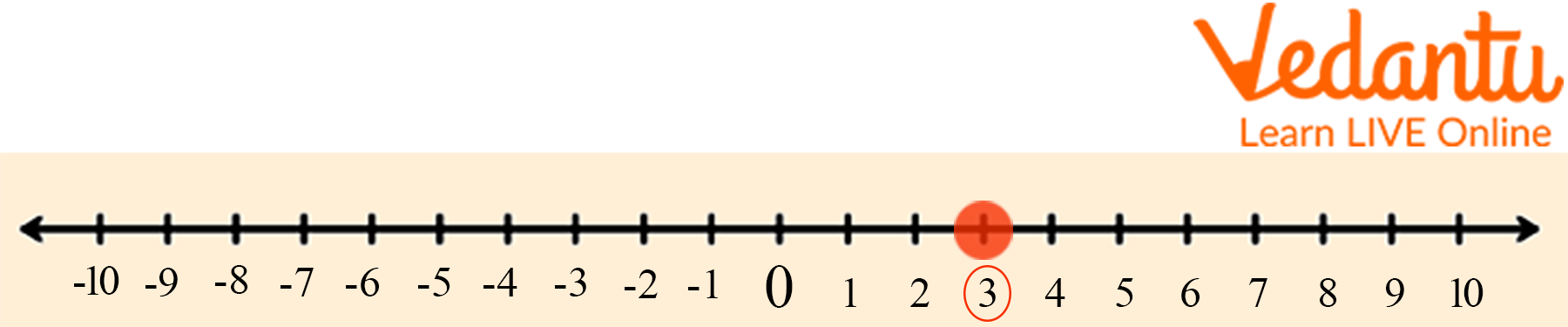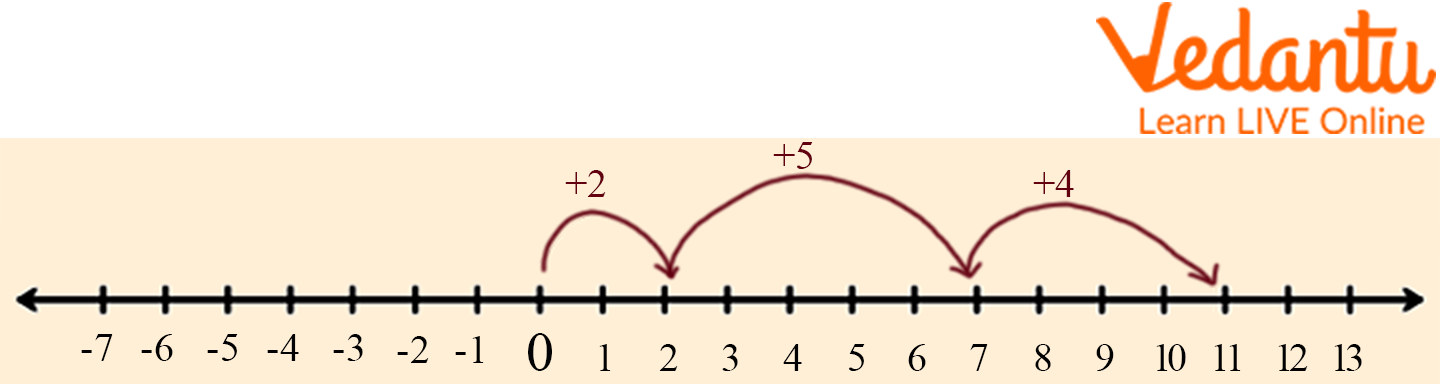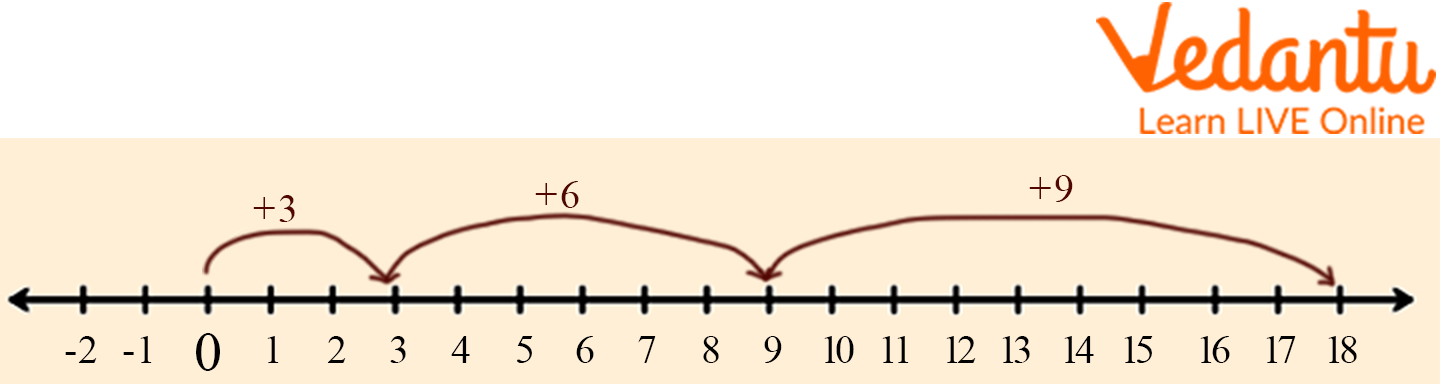Courses
Courses for Kids
Free study material
Offline Centres
More

# Add 3 Numbers on a Number LineLast updated date: 04th Dec 2023
Total views: 102.6k
Views today: 1.02k## A Brief Explanation of Add 3 Numbers on a Number Line

Numbers are important in mathematics. They help us understand and solve problems. Numbers on a number line are also important because they help us visualise how numbers increase or decrease. Numbers on a number can be added together to make bigger numbers or subtracted from each other to make smaller ones.

Additionally, it serves as a tool for teaching kids how to add using a number line. They are better able to understand how the numbers are related. They can also use it to understand how addition works in general. Kids can learn about numbers by using the number line.

## What is Number Line?

A visual representation of numbers on a straight line drawn either horizontally or vertically is known as a number line. Writing down numbers on a number line makes it easy for us to compare them and perform basic arithmetic operations. Zero (0) is the origin of a number line. The numbers to the left of 0 are negative, and those to the right of 0 are all positive numbers. So, we can say that on a number line, as we move towards the right, the value of numbers increases. This means that the numbers on the right are larger than those on the left. For example, 3 comes to the right of 1, so 3 > 1. Observe the horizontal number line given below.Number 3 on the number line

## How to Place a Number on Number Line?

We use the following steps to draw a line or plot a number on it.

1. Draw a horizontal line with arrows on both ends. (You can also draw a vertical number line)

1. Choose a scale depending on the given number. For example, if you have to plot 5, you can choose a scale of 1. If you have to plot 50, you can choose a scale of either 5, 10, or 25. (Determining an appropriate scale depends on the factors of the given number)

1. Mark the points at equal intervals. So, if you have chosen 1 as the scale, mark 0, 1, 2, 3, 4, and so on.

1. Locate the given number. Highlight that point with a circle.

### Below is an example showing the number line:Number line

For Example: Add 1 + 2

Let us look into the steps below to understand addition on a number line.Step 1: Consider the first number (1) as the starting point on the number line. To add 1 + 2, mark 1 on the number line.

Step 2: Now, from the first number, jump by the number of units equivalent to the second number towards the right. This is because the values on a number increase as we move towards the right-hand side. In this case, we are adding 1 + 2; therefore, we will move 2 steps to the right. This will bring us to number 3.

So, 1 + 2 = 3

## Activity to Add Using the Number Line

Kids are supposed to use this activity to add three numbers on a number line.

1. Find the first number on the number line and add it to the second number on the line.

2. Add that number to the third number on the line and write down your answer in your book or on a piece of paper.

3. Your final response should be recorded in your book or on paper when you add that response back to your initial response.

In mathematics, numbers are placed on a number line. Children will practice adding using a number line in this activity.

1. Start with a blank number line and write the word “one” at the bottom of the number line.

2. Write two dots under the word “one” and then write “two” under it.

3. Write three dots under the word “two” and then write “three” under it.

4. Continue this pattern until you have reached your desired point on the number line

## Locate 3 on the Number Line

To locate 3 on the number line, first, we draw a number line and then find the number 3 on it.Locate 3 on the number line

## Solved Examples

In this activity, we will add using a number line. This is a great way to practice your additional skills and learn how to read a number line.

Example- Using a number line, add 2, 5 and 4.

Ans: Adding 2,5, and 4 using the number line gives 11.Using the number line

Example- Using the number line add 3, 6 and 9.

Ans: Adding 3,6, and 9 using the number line gives 18.Example: Add 1, 5 and 6 using the number line.

Ans: Adding 1,5 and 6 using the number line gives 12.Add 3 numbers on a number line

## Number Line Questions for Practice

Here are some number line questions, which are like this;

Q 1. 1+2+3

Ans. 6

Q 2. 4+6+3

Ans. 13

Q 3. 2+6+7

Ans. 15

## Summary

In mathematics, adding numbers on a number line is a way to visualise addition. This is a great tool for kids to learn how to add numbers and practice the basics of addition. This article discusses the different ways to help children learn to add 3 numbers on a number line. The number line is a very important tool in mathematics. The article explains how to add using a number line and then provides a few number line questions that can be solved to better understand the concept.

## FAQs on Add 3 Numbers on a Number Line

1. What benefits can come from using a number line in Mathematics for kids?

To understand the benefits of using number lines in Math, we need to understand what they are. Number lines are a visual representation of numbers that helps children learn and develop their math skills. Number lines can be used to teach addition and subtraction by simply drawing one line for each number. They also help with number recognition, the order of numbers, and other math concepts.

2. Is there a difference between a number line and a graph of numbers?

A number line is a continuous line that represents the numbers from 0 to infinity. On the other hand, a graph of numbers is a collection of points with a number representing each point.

3. How many numbers are on a number line?

A number line is a line with numbers representing the whole numbers from zero to infinity. Numbers are one of the most important concepts in mathematics. Students can use a number line to visualise how numbers behave and learn new patterns and ideas.